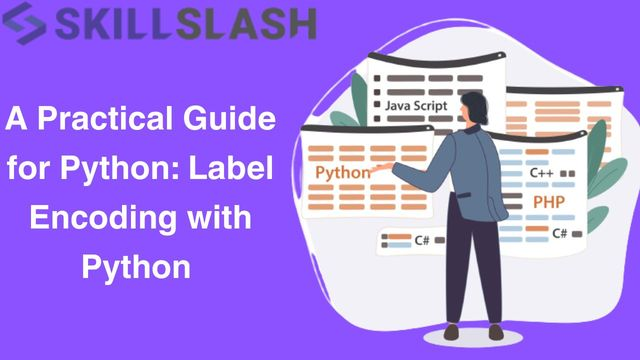News

Oct 25 @ 5:28 amA Practical Guide for Python Label Encoding with P

Introduction

If you’re a data scientist, label encoding is one of the most important tools you’ll have in your toolbox. Machine learning algorithms often need numerical inputs, and label encoding makes it easy to convert categories into integers; this way you can feed your data into a machine learning model and get your results in no time. It’s a great skill to have, especially when you’re working on real-world data with lots of categorical features.

What’s even better about label encoding is that it is quite easy to do. It is simply a matter of putting the encoder on your data and turning it into numbers. Label encoding is like a bridge between the world of data and numbers, it can be used to unlock the predictive power of data, one number at a time. Whether you're a pro or just starting out, learning how to use label encoding is a great way to get the most out of your data in Python.

What is Label Encoding ?

Label encoding is the process of converting categorical data into numerical values. It assigns a unique integer to each category in a particular feature or column. This transformation is particularly useful when working with machine learning models because most algorithms require numerical input data.

Let's dive into the steps to perform label encoding with Python:

STEP 1: Import Libraries

First, you need to import the necessary libraries. For label encoding, you can use the ‘LabelEncoder’ class from the ‘scikit-learn’ library.

 python (code sample)   from sklearn.preprocessing import LabelEncoder

STEP 2: Create Sample Data

For the sake of this example, let’s create a simple dataset with a categorical feature:

 python (code sample)   data = ['cat', 'dog', 'fish', 'dog', 'cat']

STEP 3: Initialize the LabelEncoder

Create an instance of the ‘LabelEncoder’ class

 python (code sample)   label_encoder = LabelEncoder()

STEP 4: Fit and Transform

Now, you’ll fit the label encoder to your data and transform the data to obtain encoded values.

 python (code sample)   encoded_data = label_encoder.fit_transform(data)

The ‘fit_transform’ method both fits the encoder to your data (determining the mapping of categories to integers) and transforms the data

STEP 5: View the Encoded Data

You can view the encoded data and the corresponding mapping of categories to integers as follows:

 python (code sample)   print("Original Data:", data) print("Encoded Data:", encoded_data) print("Category Mapping:", dict(zip(data, encoded_data)))

Output:

 Original Data: ['cat', 'dog', 'fish', 'dog', 'cat'] Encoded Data: [0 1 2 1 0] Category Mapping: {'cat': 0, 'dog': 1, 'fish': 2}

As you can see above, the original categorical data has been transformed into numerical values. “cat” is represented as 0, “dog” as 1, and “fish” as 2.

Using Label Encoding in Real-World Data

In real-world situations, it is common to work with datasets that contain multiple elements and multiple categories. Label encoding is capable of being used for particular columns, and may need to be combined with other preprocessing methods, such as one-hot encoding, for more intricate cases.

Here's an example of label encoding with a dataset loaded from a CSV file:

 import pandas as pd from sklearn.preprocessing import LabelEncoder   # Load the dataset data = pd.read_csv('your_data.csv')   # Initialize the label encoder label_encoder = LabelEncoder()   # Apply label encoding to a specific column data['category_column'] = label_encoder.fit_transform(data['category_column'])

Conclusion

In Python, label encoding is one of the most important techniques for handling categorical data. It enables you to transform categorical variables to numerical format, which makes them suitable for Machine Learning (ML) models.

However, it is important to note that label encoding should be used with caution, especially when dealing with features with a high number of categories. The reason is that label encoding introduces ordinality into the data, which does not exist in Python. Always think about the type of data you are dealing with and choose the right encoding method accordingly.

25 Views